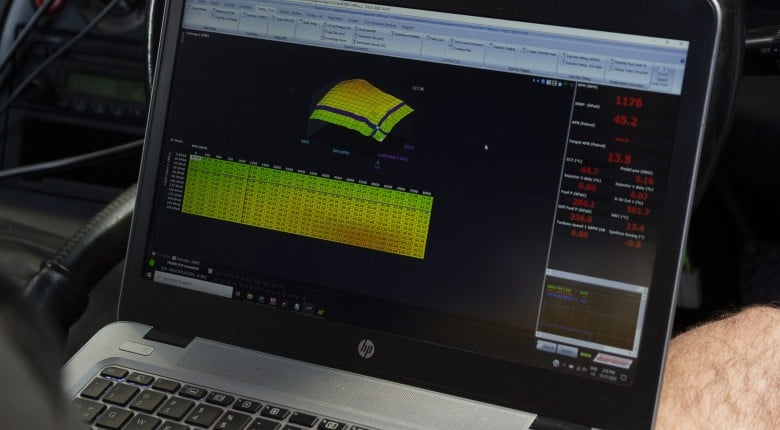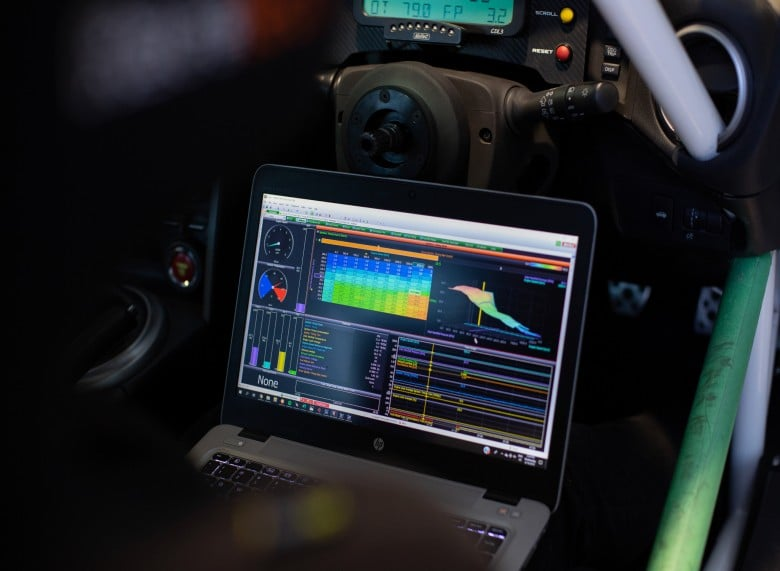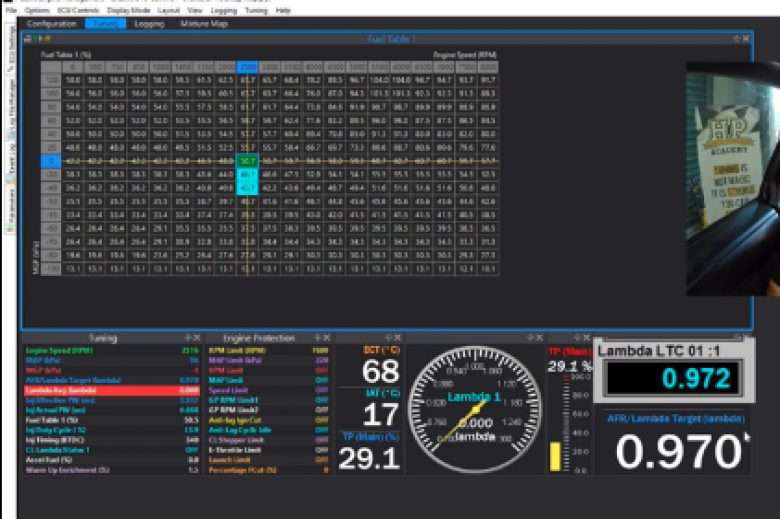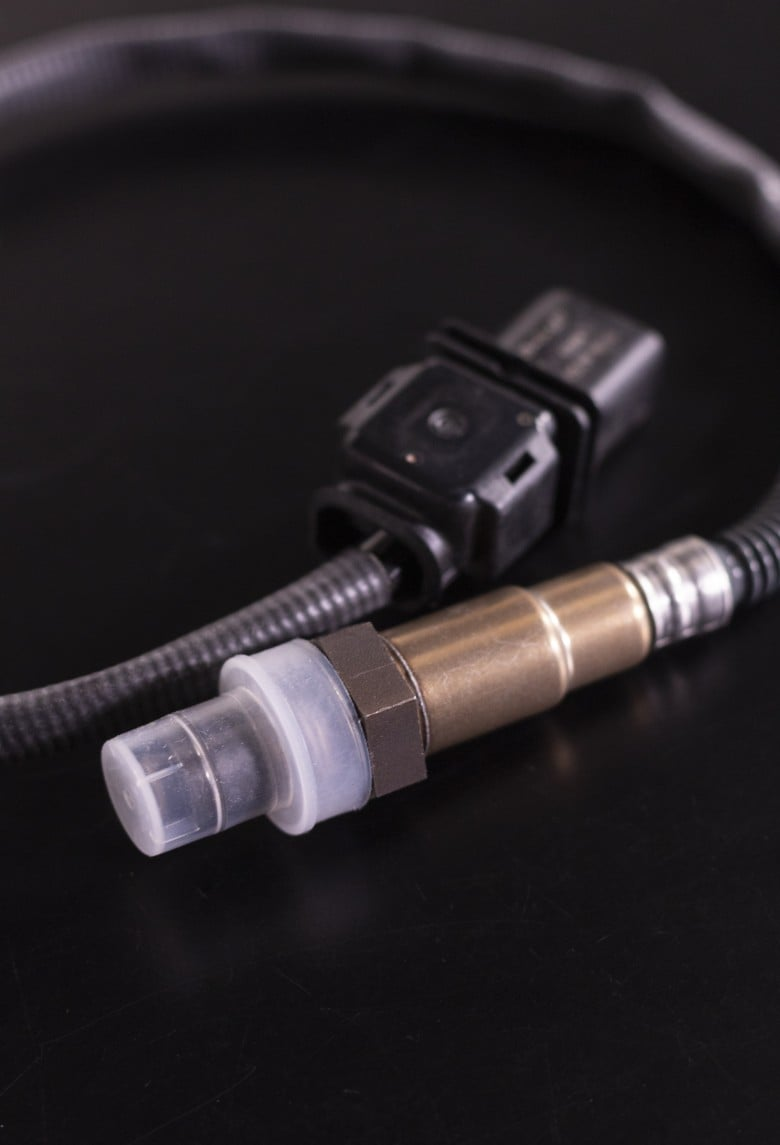As tuners, the two most common units we will use to describe air-fuel ratio are AFR and Lambda and it's important to understand the difference. The fundamental difference is that AFR describes the ratio between the mass of oxygen and fuel, while Lambda on the other hand describes the ratio between the actual AFR and the stoichiometric AFR.

Before we get too deep into this, make sure you grab your Understanding Air Fuel Ratio 'cheat sheet' here which covers the formulas mentioned below and much more.

### What Is Lambda?

In basic terms, Lambda describes how much richer or leaner the air-fuel ratio is compared to stoichiometric as a percentage. In units of Lambda, a number of 1.0 means that the engine is running at a stoichiometric AFR. Numbers larger than 1 represent a lean mixture or one with an excess of oxygen, while numbers less than 1 represent a rich mixture or one with an excess of fuel. It might sound like a subtle difference, but the fact that Lambda gives us a value relative to stoichiometric is very important, particularly if you are often dealing with fuels that have different stoichiometric air fuel ratios, and we'll explain why that can make using AFR harder by comparison below.### How Does Lambda Work And Convert To AFR?

Let’s look at an example so you can see how the different units work. Let’s say we have normal pump gasoline with a stoichiometric air-fuel ratio of 14.7:1, and we are running the engine with a measured air-fuel ratio of 12.5:1. If we were displaying the air-fuel ratio in units of Lambda instead, we would get a value of 0.85 which is simply 12.5:1/14.7:1.

If on the other hand we were measuring a Lambda value of 1.05 and we want to see what this is in units of AFR, we can just multiply the Lambda value by the stoichiometric value. In this case, 1.05*14.7 gives us the answer 15.4, which means 15.4:1 (15.4 to 1 as explained along with much more in the Understanding AFR course just in case you weren't sure about that part!).### So what is better? AFR or Lambda?

Well, that comes down to your own opinion and experience, but we here at HPA prefer to use the Lambda scale for a few reasons.

First off, we prefer Lambda because it is much easier for a tuner to look at the Lambda value and figure out at a glance how rich or lean they are. For example, if we’re running at 0.85 Lambda, I can subtract 0.85 from 1.00 which gives me 0.15, which means I’m running 15% richer than stoichiometric. In reality, though you don’t need a calculator and I know that 0.90 for example is 10% richer or 1.03 is 3% leaner than stoichiometric.

Great, but why does that even matter? Well, this can speed up your tuning by allowing you to make fast, accurate percentage adjustments to your fuel table to correct errors without the need for a calculator. Of course, the same process can be applied if you chose to tune in units of AFR, but unless you are great with math, the percentages aren’t so obvious to you at a glance.

Another advantage if you are tuning on fuels with different stoichiometric air-fuel ratios is that with the Lambda scale, often our target Lambda doesn’t change much. For example, if we have a turbocharged engine and switch from pump fuel to E85, we could safely target a Lambda value of 0.80 at 1 bar of boost on either fuel. If we were tuning in units of AFR though our target would change significantly. On pump fuel, our target AFR would be 0.80 multiplied by 14.7 which gives us a target of 11.8:1. E85 has a stoichiometric ratio of 9.8:1 though so targeting the same Lambda value of 0.80 would result in a target mixture of 0.8 multiplied by 9.8 which is 7.8:1.

With the above in mind in our opinion, the major advantage to the Lambda scale is that it avoids confusion when tuning on popular ethanol blends. Most tuners quote AFR targets on ethanol using the gasoline stoichiometric value, which isn't technically correct but seems to be pretty universal. This can be confusing though and sticking to Lambda avoids any potential for confusion and mistakes.### Conclusion

Lambda is a tool that always shows where our fuelling is relative to the stoichiometric AFR of a specific fuel, thus allowing tuners to make quick, accurate calculations.

Failing to understand how the stoichiometric ratio of a particular fuel can affect your target AFR can be potentially disastrous (that is why we must tune to get it right!) and using Lambda helps eliminate some risk of human error with the upside of also being faster. If you want to get a greater understanding of AFR, check out the Understanding AFR course or why not even take a free EFI tuning lesson and learn about some ignition tuning too.